# TensorFlow 基礎¶

• Python 基本操作 （賦值、程式分支及控制流程、使用 import 導入套件）；

• NumPy ，Python 下常用的科學計算套件。TensorFlow 與之結合緊密；

• 向量矩陣 運算（矩陣的加減法、矩陣與向量相乘、矩陣與矩陣相乘、矩陣的轉置等。測試題：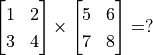）；

• 函數的導數多元函數推導 （測試題：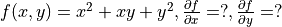）；

• 線性迴歸

• 梯度下降方法 求函數的局部最小值。

## TensorFlow 1+1¶

import tensorflow as tf


TensorFlow 使用 **張量**（Tensor）作為資料的基本單位。TensorFlow 的張量在概念上等同於多維陣列，我們可以使用它來描述數學中的純量（0 維陣列）、向量（1 維陣列）、矩陣（2 維陣列）等各種量，範例如下：

# 定義一個隨機數（純量）
random_float = tf.random.uniform(shape=())

# 定義一個有2個元素的零向量
zero_vector = tf.zeros(shape=(2))

# 定義兩個2×2的常量矩陣
A = tf.constant([[1., 2.], [3., 4.]])
B = tf.constant([[5., 6.], [7., 8.]])


# 查看矩陣A的形狀、類型和值
print(A.shape)      # 輸出(2, 2)，即矩陣的長和寬均為2
print(A.dtype)      # 輸出<dtype: 'float32'>
print(A.numpy())    # 輸出[[1. 2.]
#      [3. 4.]]


TensorFlow 的大多數 API 函數會根據輸入的值自動推斷張量中元素的類型（一般預設為 tf.float32 ）。不過你也可以通過加入 dtype 參數來自行指定類型，例如 zero_vector = tf.zeros(shape=(2), dtype=tf.int32) 將使得張量中的元素類型均為整數。張量的 numpy() 方法是將張量的值轉換為一個 NumPy 陣列。

TensorFlow 裡有大量的運算函數（Operation），使得我們可以將已有的張量進行運算後得到新的張量。範例如下：

C = tf.add(A, B)    # 計算矩陣A和B的和
D = tf.matmul(A, B) # 計算矩陣A和B的乘積


tf.Tensor(
[[ 6.  8.]
[10. 12.]], shape=(2, 2), dtype=float32)
tf.Tensor(
[[19. 22.]
[43. 50.]], shape=(2, 2), dtype=float32)


## 自動推導機制¶

import tensorflow as tf

x = tf.Variable(initial_value=3.)
with tf.GradientTape() as tape:     # 在 tf.GradientTape() 的上下文內，所有計算步驟都會被記錄以用於推導
y = tf.square(x)


tf.Tensor(9.0, shape=(), dtype=float32)
tf.Tensor(6.0, shape=(), dtype=float32)


tf.GradientTape() 是一個自動推導的記錄器。只要進入了 with tf.GradientTape() as tape 的上下文環境，則在該環境中計算步驟都會被自動記錄。比如在上面的範例中，計算步驟 y = tf.square(x) 即被自動記錄。離開上下文環境後，記錄將停止，但記錄器 tape 依然可用，因此可以通過 y_grad = tape.gradient(y, x) 求張量 y 對變數 x 的導數。

X = tf.constant([[1., 2.], [3., 4.]])
y = tf.constant([[1.], [2.]])
w = tf.Variable(initial_value=[[1.], [2.]])
b = tf.Variable(initial_value=1.)
with tf.GradientTape() as tape:
L = tf.reduce_sum(tf.square(tf.matmul(X, w) + b - y))
w_grad, b_grad = tape.gradient(L, [w, b])        # 計算L(w, b)關於w, b的偏導數


tf.Tensor(125.0, shape=(), dtype=float32)
tf.Tensor(
[[ 70.]
[100.]], shape=(2, 1), dtype=float32)
tf.Tensor(30.0, shape=(), dtype=float32)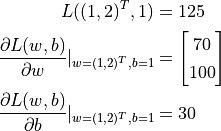## 基礎範例：線性回歸¶

 年份 2013 2014 2015 2016 2017 房價 12000 14000 15000 16500 17500

import numpy as np

X_raw = np.array([2013, 2014, 2015, 2016, 2017], dtype=np.float32)
y_raw = np.array([12000, 14000, 15000, 16500, 17500], dtype=np.float32)

X = (X_raw - X_raw.min()) / (X_raw.max() - X_raw.min())
y = (y_raw - y_raw.min()) / (y_raw.max() - y_raw.min())


• 初始化自變數為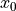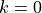• 疊代進行下列步驟直到滿足收斂條件：

• 求函数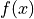關於自變數的梯度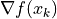• 更新自變數：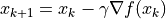。這裡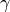是學習率（也就是梯度下降一次邁出的“步長”大小）

•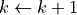### NumPy 下的線性回歸¶

a, b = 0, 0

num_epoch = 10000
learning_rate = 5e-4
for e in range(num_epoch):
# 手動計算損失函數關於自變數（模型參數）的梯度
y_pred = a * X + b
grad_a, grad_b = 2 * (y_pred - y).dot(X), 2 * (y_pred - y).sum()

# 更新參數
a, b = a - learning_rate * grad_a, b - learning_rate * grad_b

print(a, b)


• 經常需要人工推導求函數關於參數的偏導數。如果是簡單的函數或許還好，但一旦函數的形式變得複雜（尤其是深度學習模型），人工推導的過程將變得非常痛苦，甚至不可行。

### TensorFlow下的線性回歸¶

TensorFlow的 即時執行模式 5 與上述 NumPy 的運行方式十分類似，然而提供了更快速的運算（GPU 支援）、自動推導、優化器等一系列對深度學習非常重要的功能。以下展示了如何使用 TensorFlow 計算線性回歸。可以注意到，程式的結構和前述 NumPy 的實現非常類似。這裡，TensorFlow 幫助我們做了兩件重要的工作：

• 使用 tape.gradient(ys, xs) 自動計算梯度；

• 使用 optimizer.apply_gradients(grads_and_vars) 自動更新模型參數。

X = tf.constant(X)
y = tf.constant(y)

a = tf.Variable(initial_value=0.)
b = tf.Variable(initial_value=0.)
variables = [a, b]

num_epoch = 10000
optimizer = tf.keras.optimizers.SGD(learning_rate=5e-4)
for e in range(num_epoch):
with tf.GradientTape() as tape:
y_pred = a * X + b
loss = tf.reduce_sum(tf.square(y_pred - y))
# TensorFlow自動計算損失函數關於自變數（模型參數）的梯度
# TensorFlow自動根據梯度更新參數

print(a, b)


Python的 zip() 函數

zip() 函數是 Python 的內建函數。用自然語言描述這個函數的功能很繞口，但如果舉個例子就很容易理解了：如果 a = [1, 3, 5]b = [2, 4, 6]，那麼 zip(a, b) = [(1, 2), (3, 4), ..., (5, 6)] 。即 “將可疊代的對象作為參數，將對象中對應的元素打包成一個個元組，然組合後返回由這些組合組成的列表”，和我們日常生活中拉上拉鏈（zip）的操作有異曲同工之妙。在 Python 3 中， zip() 函數返回的是一個 zip 對象，本質上是一個生成器(Generator)，需要呼叫 list() 來將生成器轉換成列表。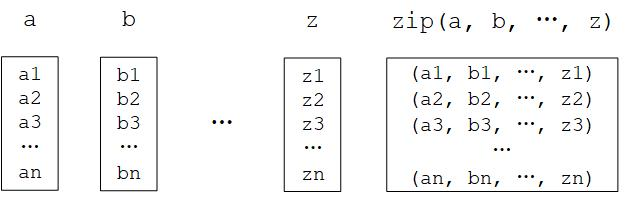Python的 zip() 函數圖示

1

Python 中可以使用整數後加小數點表示將該整數定義為浮點數類型。例如 3. 代表浮點數 3.0

2

3

4

5